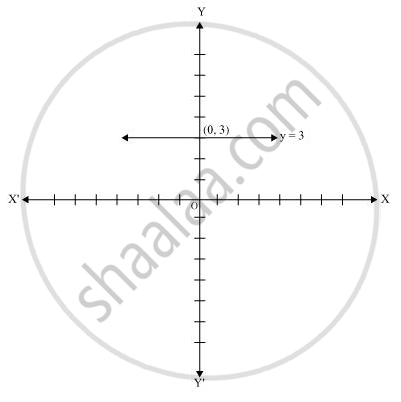# Give the geometric representations of y = 3 as an equation in two variables. - Mathematics

Give the geometric representations of y = 3 as an equation in two variables.

#### Solution

In two variables, y = 3 represents a straight line passing through point (0, 3) and parallel to x-axis. It is a collection of all points of the plane, having their y-coordinate as 3.Concept: Equations of Lines Parallel to the X-axis and Y-axis
Is there an error in this question or solution?

#### APPEARS IN

NCERT Class 9 Maths
Chapter 4 Linear Equations in two Variables
Exercise 4.4 | Q 1.2 | Page 77